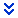# Analysis with Correlation and Regression presentation

### Description

Determine and interpret the linear correlation coefficient, and use linear regression to find a best fit line for a scatter plot of the data and make predictions.

## Scenario

According to the U.S. Geological Survey (USGS), the probability of a magnitude 6.7 or greater earthquake in the Greater Bay Area is 63%, about 2 out of 3, in the next 30 years. In April 2008, scientists and engineers released a new earthquake forecast for the State of California called the Uniform California Earthquake Rupture Forecast (UCERF).

As a junior analyst at the USGS, you are tasked to determine whether there is sufficient evidence to support the claim of a linear correlation between the magnitudes and depths from the earthquakes. Your deliverables will be a PowerPoint presentation you will create summarizing your findings and an excel document to show your work.

Concepts Being Studied

• Correlation and regression
• Creating scatterplots
• Constructing and interpreting a Hypothesis Test for Correlation using r as the test statistic

You are given a spreadsheetthat contains the following information:

• Magnitude measured on the Richter scale
• Depth in km

Using the spreadsheet, you will answer the problems below in a PowerPoint presentation.

## What to Submit

The PowerPoint presentation should answer and explain the following questions based on the spreadsheet provided above.

• Slide 1: Title slide
• Slide 2: Introduce your scenario and data set including the variables provided.
• Slide 3: Construct a scatterplot of the two variables provided in the spreadsheet. Include a description of what you see in the scatterplot.
• Slide 4: Find the value of the linear correlation coefficient r and the critical value of r using ? = 0.05. Include an explanation on how you found those values.
• Slide 5: Determine whether there is sufficient evidence to support the claim of a linear correlation between the magnitudes and the depths from the earthquakes. Explain.
• Slide 6: Find the regression equation. Let the predictor (x) variable be the magnitude. Identify the slope and the y-intercept within your regression equation.
• Slide 7: Is the equation a good model? Explain. What would be the best predicted depth of an earthquake with a magnitude of 2.0? Include the correct units.
• Slide 8: Conclude by recapping your ideas by summarizing the information presented in context of the scenario.

Explanation & Answer length: 8 Slides Presentation.

MAG 0.70 0.74 0.64 0.39 0.70 2.20 1.98 0.64 1.22 0.20 1.64 1.32 2.95 0.90 1.76 1.01 1.26 0.00 0.65 1.46 1.62 1.83 0.99 1.56 0.40 1.28 0.83 1.34 0.54 1.25 0.92 1.00 0.79 0.79 1.44 1.00 2.24 2.50 1.79 1.25 1.49 0.84 1.42 1.00 1.25 1.42 1.35 0.93 0.40 1.39

DEPTH 7.2 2.2 13.9 15.5 3.0 2.4 14.4 5.7 6.1 7.1 17.2 8.7 9.3 12.3 7.0 7.4 17.1 8.8 5.0 19.1 12.7 4.7 6.8 6.0 14.6 4.9 19.1 9.9 16.1 4.6 4.9 16.3 14.0 4.2 5.4 5.9 15.6 7.7 16.4 15.4 4.9 8.1 7.5 14.1 11.1 16.0 5.5 7.3 3.1 6.0

Do you have a similar assignment and would want someone to complete it for you? Click on the ORDER NOW option to get instant services at EssayBell.com